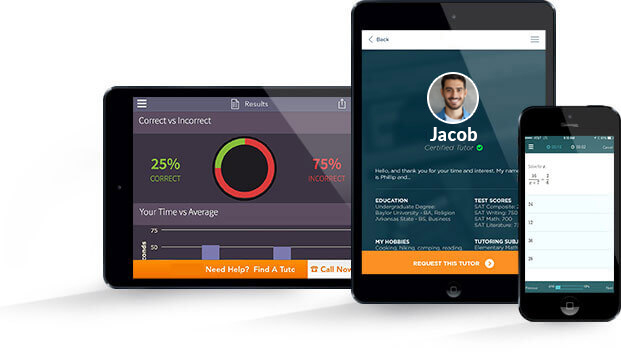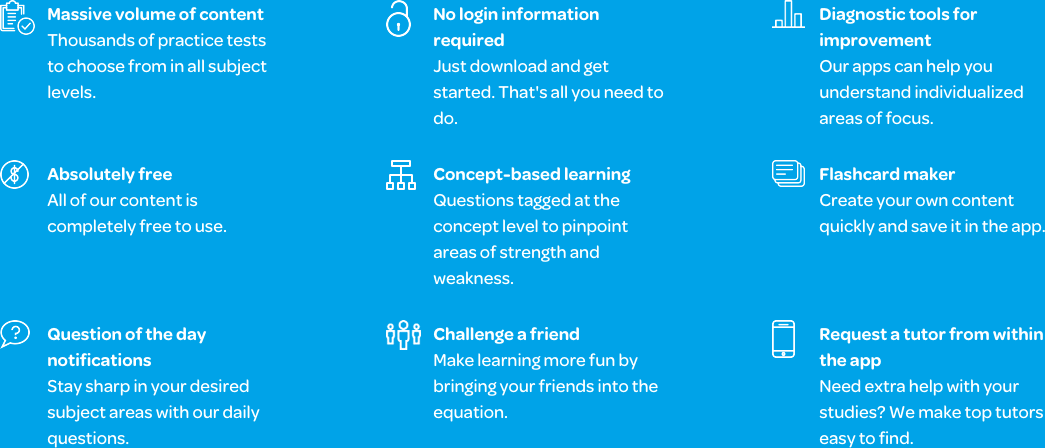# Varsity Tutors Intermediate Geometry mobile app### The Varsity Tutors Intermediate Geometry Mobile App

Performing well in an intermediate geometry course requires a good understanding of graphing and functions. If you are having trouble finding the area or perimeter of a circle, figuring out the volume of a cone or sphere, or remembering all the many formulas used in geometry, some help outside the classroom is probably what you need.

That’s where Varsity Tutor’s free Intermediate Geometry App for iOS and Android devices comes in. The app will take you through the entire course, testing what you know and helping you learn at the same time.

Though it’s not usually a required high school course, taking intermediate geometry has been proven to lead to higher scores on national standardized tests such as the ACT, SAT, and the NAEP (National Association for Educational Progress) mathematics assessment.
The curriculum for intermediate geometry is comprised of a combination of beginning and advanced geometry concepts. Topics covered include coordinate geometry, plane geometry, and solid geometry.

In the beginning of the course you will study coordinate geometry, where you will learn how to find the equation of a circle using the distance formula, given the radius, diameter, or other points on the graph. You’ll also work with lines, using the distance and midpoint formulas, to find the length, endpoint, or midpoints of lines or segments. Also covered will be parallel, perpendicular, and tangent lines – how to find out which kind of lines they are, the equation for the line, and the slope of the line.

Next, your focus will turn to plane geometry, this time focusing on concepts such as how to find the length of a chord, the angle and area of a sector, the length of an arc, and the percentage of a sector from the angle. You will learn how to find the angle, area, perimeter, length of a side, and length of a diagonal for shapes such as triangles, hexagons, and pentagons.

Finally, you will learn about solid geometry, where you’ll be working with 3D shapes like cubes, cylinders, prisms, and spheres. In addition to finding many of the same characteristics for 3D shapes as you would for 2D shapes (for example, the diagonal), you will also learn how to find more complex characteristics, such as a shape’s volume and surface area.

The free Varsity Tutors Intermediate Geometry app for iPhone, iPad, and Android devices has many tools for learners. First, you could take a Full-Length Practice Test to determine your strengths and any areas that need work. This will allow you to focus your studies when you move on to the Learn by Concept section and flashcards.

If you need to work specifically on kites or rhombuses, you can click right to that section and focus your learning on that topic. The app does more than test what you already know, though; it also helps you learn. After each question, the app gives a detailed explanation for how to get to the correct answer. This way, you can see not only what the right answer is, but how to reach it. If test-taking itself is a challenge for you, the app provides practice tests with content similar to tests you would take in your school’s intermediate geometry class.

Download the free Varsity Tutors Intermediate Geometry app for iPhone, iPad, and Android today, and begin taking advantage of these valuable learning supplements today.Intermediate Geometry is a high school course that teaches you the basics of plane geometry, coordinate geometry, and solid geometry. You will learn all about two- and three-dimensional shapes, and how to create, manipulate, and work with them. Ultimately, you will be able to use math to calculate the area and volume of shapes, and their perimeters as well. Other challenging calculations include finding the diagonal of a shape’s surface area. You will work with tetrahedrons and cones, trapezoids and rhombuses, and other familiar shapes. As you will see, there is more to these shapes than meets the eye.

Graphing is a big part of Intermediate Geometry. It moves on from graphing linear functions and ordered pairs to graphing two-step inequalities and complex numbers. Quadratic functions, exponentials, logarithms, and inverse variations may factor in. The problem solving can get quite intense, which gives many students anxiety. Prepare yourself ahead of time. Things are about to get interesting, but Intermediate Geometry can be a fascinating experience in analyzing complex shapes in ways you probably haven’t yet imagined.

Intermediate Geometry starts with the basics of geometry. This stage covers the essentials of coordinate geometry, which involves working with points on a graph, which you’ll be plotting in no time. Each point is found by locating its values on the x and y axes. Once you plot points, you can draw lines and identify their segments. Soon you’ll be working with parallel and perpendicular lines, tangent lines, and the x and y intercept. The course content at this stage isn’t limited to straight lines. You’ll also be working with curves, and will need to perform calculations using the equation of a curve. Gaining knowledge of working with different aspects of a shape will enable you to find lengths, angles, slopes, and other measurements of more complex shapes later on.

You will focus on the relationships between parallel and perpendicular lines. Using formulas and theorems you will learn during the course, you can actually calculate angles to determine the status of a line, which applies to complete shapes such as triangles, which you will work with in detail. You will classify and measure angles of isosceles and equilateral triangles and apply the Pythagorean Theorem. This relates the three sides of the shape, stating the square of the hypotenuse is equal to the sum of the squares of the measurements of both other sides. The hypotenuse is the side directly opposite the right angle. Other equations include the distance formula, an aspect of analytical geometry that measures the distance between two points.

These concepts help prepare you for the next topic in Intermediate Geometry – quadrilaterals. A quadrilateral is a polygon that has four sides and four corners. It can take many forms, including a parallelogram that has two pairs of parallel sides. The opposing sides are the same length, so you’ll see familiar shapes such as a square, rectangle, or a rhombus. In all of these, you’ll have to find angles, lengths, and perimeters, or determine if two or more shapes are similar. A kite is a shape in which two pairs of adjacent sides measure the same length. The typical format is a diagonal segment that divides the shape into congruent triangles.

The next section of Intermediate Geometry is all about circles. You know about pi by now, but will learn about the parts of a circle, tangential properties, and arcs and central angles. Additional equations will be learned, including the ratio of a circle’s circumference to its diameter, and the area enclosed. You will also learn how to use a circle in relation to a coordinate system and find and measure tangent lines through specific points. Chords will be covered as well. Each one is a straight line segment with endpoints that lie on the edge of the circle. They don’t go straight through the center, which challenges your knowledge of trigonometry to make inferences and calculations based on what you also know about triangles and circles. As you move forward, these concepts will be revisited, so it’s important to work to understand these concepts. Mastering concepts such as sine, cosine, and tangent ratio will prove useful further on.

Intermediate Geometry covers the concepts of surface area and volume as well. You’ll get a synopsis of solid figures and lessons on surface area in relation to prisms and cylinders, as well as pyramids and cones. For example, you know pi is approximately 3.14 and is the radius of a circle. A sphere’s radius is the same, but you have to multiply four times pi times the radius squared. For a cube, it’s six times the side squared. You’ll also learn and follow specific formulas for cuboids, triangular prisms, cylinders, and pyramids. The ability to find diagonals in a three-dimensional prism requires practicing formulas, as does finding the length of an edge or the surface area or volume.

Problem solving is a major part of Intermediate Geometry, so you will have to call on everything you’ve learned in math so far. As with any mathematics discipline, it involves a lot of practice. Plus, geometry and algebra go hand in hand. Knowing the common formulas and symbols will give you an advantage in grasping the concepts and understanding how to work with each shape. There’s much vocabulary to know as well. With angles alone, you’ll need to know acute, right, obtuse, and straight angles, and this skill set advances to complimentary, supplementary, and adjacent and vertical angles. Intermediate Geometry can be a mental workout, but with some patience, you can acquire skills that will be useful later on.

Acing the course means you’ll be eligible for more advanced courses and areas of study in the next phase of your academic life. The Intermediate Geometry you learn in high school can have an impact on your college and work career, whether you just want to meet basic requirements or prove your skills to a prospective college. With Intermediate Geometry, you are building a solid foundation for your academic future.